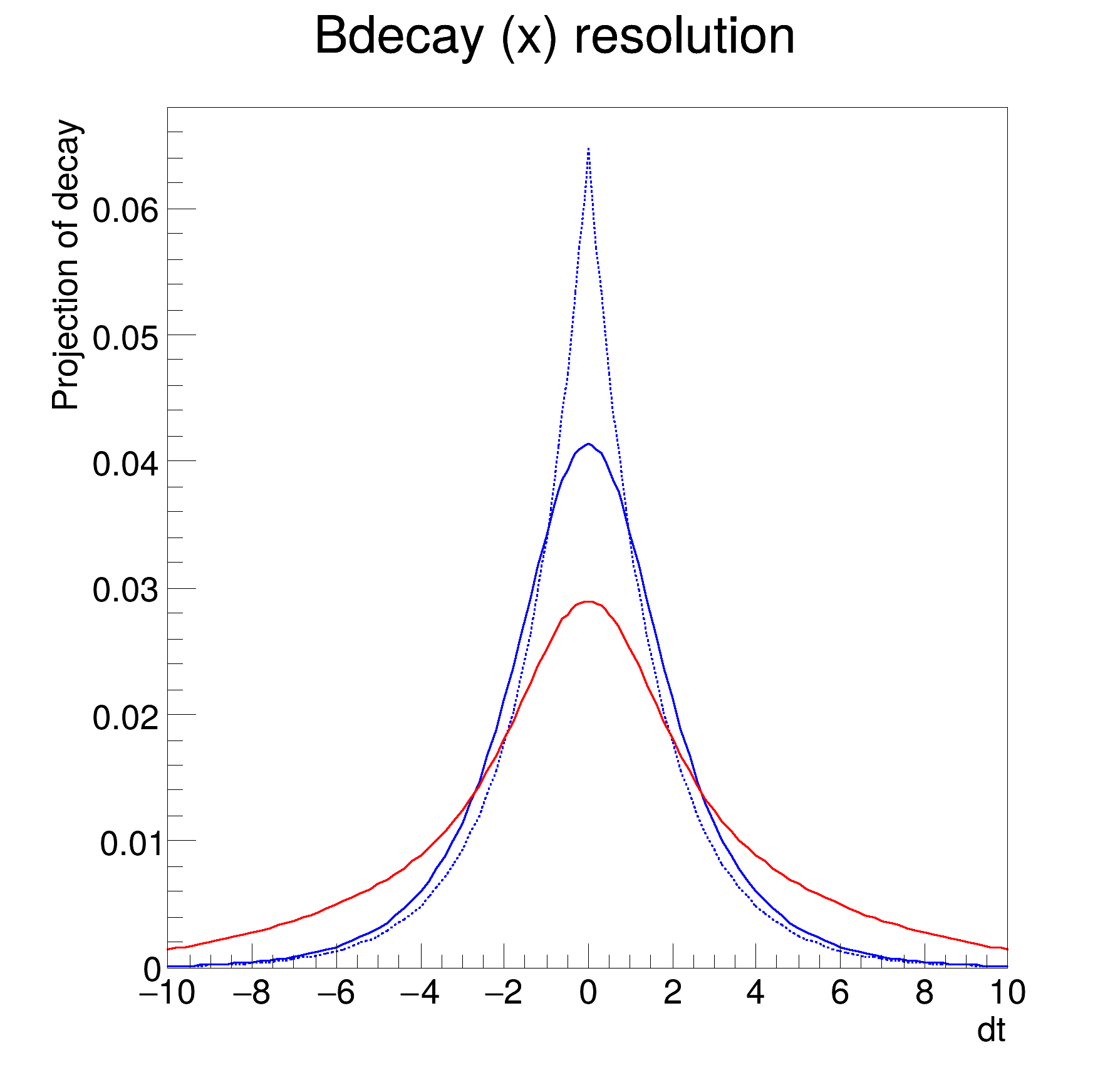ROOT   Reference Guiderf209_anaconv.C File Reference

## Detailed DescriptionAddition and convolution: decay function pdfs with optional B physics effects (mixing and CP violation)

that can be analytically convolved with e.g. Gaussian resolution functions

pdf1 = decay(t,tau) (x) delta(t)
pdf2 = decay(t,tau) (x) gauss(t,m,s)
pdf3 = decay(t,tau) (x) (f*gauss1(t,m1,s1) + (1-f)*gauss2(t,m1,s1))
#define f(i)
Definition: RSha256.hxx:104
#define s1(x)
Definition: RSha256.hxx:91
Double_t x[n]
Definition: legend1.C:17
static constexpr double s
static constexpr double gauss
TMarker m
Definition: textangle.C:8#include "RooRealVar.h"
#include "RooDataSet.h"
#include "RooGaussModel.h"
#include "RooTruthModel.h"
#include "RooDecay.h"
#include "RooPlot.h"
#include "TCanvas.h"
#include "TAxis.h"
#include "TH1.h"
using namespace RooFit;
{
// B - p h y s i c s p d f w i t h t r u t h r e s o l u t i o n
// ---------------------------------------------------------------------
// Variables of decay pdf
RooRealVar dt("dt", "dt", -10, 10);
RooRealVar tau("tau", "tau", 1.548);
// Build a truth resolution model (delta function)
RooTruthModel tm1("tm", "truth model", dt);
// Construct decay(t) (x) delta(t)
RooDecay decay_tm("decay_tm", "decay", dt, tau, tm1, RooDecay::DoubleSided);
// Plot pdf (dashed)
RooPlot *frame = dt.frame(Title("Bdecay (x) resolution"));
decay_tm.plotOn(frame, LineStyle(kDashed));
// B - p h y s i c s p d f w i t h G a u s s i a n r e s o l u t i o n
// ----------------------------------------------------------------------------
// Build a gaussian resolution model
RooRealVar bias1("bias1", "bias1", 0);
RooRealVar sigma1("sigma1", "sigma1", 1);
RooGaussModel gm1("gm1", "gauss model 1", dt, bias1, sigma1);
// Construct decay(t) (x) gauss1(t)
RooDecay decay_gm1("decay_gm1", "decay", dt, tau, gm1, RooDecay::DoubleSided);
// Plot pdf
decay_gm1.plotOn(frame);
// B - p h y s i c s p d f w i t h d o u b l e G a u s s i a n r e s o l u t i o n
// ------------------------------------------------------------------------------------------
// Build another gaussian resolution model
RooRealVar bias2("bias2", "bias2", 0);
RooRealVar sigma2("sigma2", "sigma2", 5);
RooGaussModel gm2("gm2", "gauss model 2", dt, bias2, sigma2);
// Build a composite resolution model f*gm1+(1-f)*gm2
RooRealVar gm1frac("gm1frac", "fraction of gm1", 0.5);
RooAddModel gmsum("gmsum", "sum of gm1 and gm2", RooArgList(gm1, gm2), gm1frac);
// Construct decay(t) (x) (f*gm1 + (1-f)*gm2)
RooDecay decay_gmsum("decay_gmsum", "decay", dt, tau, gmsum, RooDecay::DoubleSided);
// Plot pdf (red)
decay_gmsum.plotOn(frame, LineColor(kRed));
// Draw all frames on canvas
new TCanvas("rf209_anaconv", "rf209_anaconv", 600, 600);
frame->GetYaxis()->SetTitleOffset(1.6);
frame->Draw();
}
@ kRed
Definition: Rtypes.h:66
@ kDashed
Definition: TAttLine.h:48
RooAddModel is an efficient implementation of a sum of PDFs of the form.
RooArgList is a container object that can hold multiple RooAbsArg objects.
Definition: RooArgList.h:22
Single or double sided decay function that can be analytically convolved with any RooResolutionModel ...
Definition: RooDecay.h:22
@ DoubleSided
Definition: RooDecay.h:25
Class RooGaussModel implements a RooResolutionModel that models a Gaussian distribution.
Definition: RooGaussModel.h:25
A RooPlot is a plot frame and a container for graphics objects within that frame.
Definition: RooPlot.h:43
static RooPlot * frame(const RooAbsRealLValue &var, double xmin, double xmax, Int_t nBins)
Create a new frame for a given variable in x.
Definition: RooPlot.cxx:239
TAxis * GetYaxis() const
Definition: RooPlot.cxx:1279
void Draw(Option_t *options=nullptr) override
Draw this plot and all of the elements it contains.
Definition: RooPlot.cxx:652
RooRealVar represents a variable that can be changed from the outside.
Definition: RooRealVar.h:40
RooTruthModel is an implementation of RooResolution model that provides a delta-function resolution m...
Definition: RooTruthModel.h:21
virtual void SetTitleOffset(Float_t offset=1)
Set distance between the axis and the axis title.
Definition: TAttAxis.cxx:298
The Canvas class.
Definition: TCanvas.h:23
RooCmdArg LineColor(Color_t color)
RooCmdArg LineStyle(Style_t style)
The namespace RooFit contains mostly switches that change the behaviour of functions of PDFs (or othe...
Definition: Common.h:18
const char * Title
Definition: TXMLSetup.cxx:68
Date
July 2008

Definition in file rf209_anaconv.C.